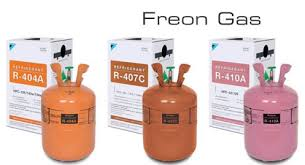ZheJiang NOF Chemical Co. Ltd.
manager@frioflor.com        +86-150-8823-9500 / +86-198-8885-5658
» » » Refrigerant Naming Rules

# Refrigerant Naming Rules

Views:5     Author:Site Editor     Publish Time: 2021-01-05      Origin:SiteThe refrigerant code is specified in the standard (ASHRAE Standard 34-67) formulated by the American Heating and Refrigeration Engineering Association in 1967. This standard numbering method is to associate the code name of the refrigerant with its species and chemical composition. As long as you know its chemical formula, you can write its code name. The code name is composed of the letter "R" and the number behind it. R stands for refrigerant (refrigerating medium) "Refrigerant" (previously F stands for Freon "Freon", and now all use the internationally recognized R to name refrigerant).

1. Inorganic compound refrigerant

Such as ammonia named: R717 (molecular formula NH3)

"7" represents inorganic compounds, and 17 is the integer part of its molecular weight.

2. Freon refrigerant

Freon is a general term for derivatives of halogen elements in saturated hydrocarbons (alkanes).

The molecular formula of saturated hydrocarbons is: CmH2m+2, when H2m+2 is partially or completely replaced by fluorine, chlorine or bromine, the resulting derivative is CmHnFxClyBrz, which is the molecular formula of Freon, and n+x+ y+z=2m+2.

For the methane system, because m=1, n+x+y+z =4

For the ethane system, because m=2, n+x+y+z=6

The code name of Freon is composed of R(m-1)(n+1)(x)B(z), if z=0, then B can be omitted, for example:

Difluoro-chloromethane, the molecular formula is CHF2Cl, m-1=0, n+1=2, x=2, z=0, so the code is R22.

Difluorodichloromethane, the molecular formula is CF2Cl2, m-1=0, n+1=1, x=2, z=0, so the code is R12.

3. Saturated hydrocarbons

The code numbering rules are the same as Freon, such as:

Methane is R50

Ethane is R170

Propane is R290

But butane is not written according to the above rules, and written as R600.

In addition, if it is an allotrope, add the letter "a" after the code or add a number to the single digit, for example, isodifluoroethane is R152a, isobutane is R600a, etc.

4. Cyclic compounds

For cyclic organic compounds, the letter "C" is added after R, and then written according to the Freon numbering rules, such as:

Hexafluorodichlorocyclobutane writing RC316

Octafluorocyclobutane writing RC318

5. Unsaturated hydrocarbons and their halogen derivatives

For this type of refrigerant, write a "1" after the R, and then write it according to the Freon numbering rules. Such as:

Ethylene is R1150

Propylene is R1270

Difluorodichloroethylene is R1112a.

6. Azeotropic refrigerant

It is composed of two or more mutually soluble single refrigerants mixed in a certain proportion at room temperature. Its properties are the same as those of a single refrigerant. It has a constant evaporation temperature under a constant pressure, and the gas and liquid phases The components are the same.

The azeotropic refrigerant stipulates in the standard that the first number after R is "5", and the two numbers after it are numbered in the order of practicality.

Such as: R500, R501, R502... R507

7. Non-azeotropic refrigerant

A solution composed of two or more single refrigerants that do not form an azeotropic solution with each other. When the solution is heated, under a certain evaporation pressure, the more volatile components evaporate in a large proportion, and the less volatile components The proportion of part evaporation is small, so the composition of the gas and liquid phases are not the same, and the temperature of the refrigerant changes during the evaporation process, and it also has similar characteristics during the condensation process.

In the refrigerant numbering standard, non-azeotropic refrigerants have not been numbered, but the numbering sequence of 400 behind R is reserved for supplementary numbering.

Such as: R400, R401, R402,...R411

Named according to the Montreal agreement: to distinguish the degree of destruction of the atmospheric ozone layer by Freon.

1. CFC (chlorofluorocarbon):

It does not contain hydrogen, public hazards, and severely damages the ozone layer, such as: R12, CFC-12, molecular formula CF2Cl2; R11, CFC11, molecular formula CFCl3.

2. HCFC (hydrochlorofluorocarbon):

Hydrogen-containing, low-pollution substances are transitional substances, such as: R22, HCFC-22, and molecular formula CHF2Cl.

3. HFC (hydrofluorocarbon):

It does not contain chlorine and is pollution-free. As a substitute, it needs to be researched and developed, such as: R134A, HFC-134, molecular formula C2H2F4.